# matrex

A blazing fast matrix library for Elixir/Erlang with C implementation using CBLAS.

View the Project on GitHub versilov/matrex

#Matrex

Fast matrix manipulation library for Elixir implemented in C native code with highly optimized CBLAS sgemm() used for matrix multiplication.

For example, vectorized linear regression is about 13 times faster, than Octave single threaded implementation.

It’s also memory efficient, so you can work with large matrices, about billion of elements in size.

Based on matrix code from https://gitlab.com/sdwolfz/experimental/-/tree/master/exlearn

## Benchmarks

#### Comparison with NumPy

2015 MacBook Pro, 2.2 GHz Core i7, 16 GB RAM

Operations are performed on 3000×3000 matrices filled with random numbers.

You can run benchmarks from the `/bench` folder with `python numpy_bench.py` and `MIX_ENV=bench mix bench` commands.

#### NumPy

``````benchmark         iterations	average time
logistic_cost()   1000         	1.23 ms/op
np.divide(A, B)   100           15.43 ms/op
sigmoid(A)        50            93.28 ms/op
np.dot(A, B)      10            196.57 ms/op
``````

#### Matrex

``````benchmark     iterations   average time
logistic_cost()      1000  1.23 ms/op (on par)
divide(A, B)         200   7.32 ms/op (~ 2× faster)
add(A, B)            200   7.71 ms/op (~ 2× faster)
sigmoid(A)           50    71.47 ms/op (23% faster)
dot(A, B)            10    213.31 ms/op (8% slower)
``````

#### Comparison with pure Elixir libraries

Slaughter of the innocents, actually.

2015 MacBook Pro, 2.2 GHz Core i7, 16 GB RAM

Dot product of 500×500 matrices

Library Ops/sec Compared to Matrex
Matrex 674.70
Matrix 0.0923 7 312.62× slower
Numexy 0.0173 38 906.14× slower
ExMatrix 0.0129 52 327.40× slower

Dot product of 3×3 matrices

Library Ops/sec Compared to Matrex
Matrex 3624.36 K
GraphMath 1310.16 K 2.77x slower
Matrix 372.58 K 9.73x slower
Numexy 89.72 K 40.40x slower
ExMatrix 35.76 K 101.35x slower

Transposing 1000x1000 matrix

Library Ops/sec Compared to Matrex
Matrex 428.69
ExMatrix 9.39 45.64× slower
Matrix 8.54 50.17× slower
Numexy 6.83 62.80× slower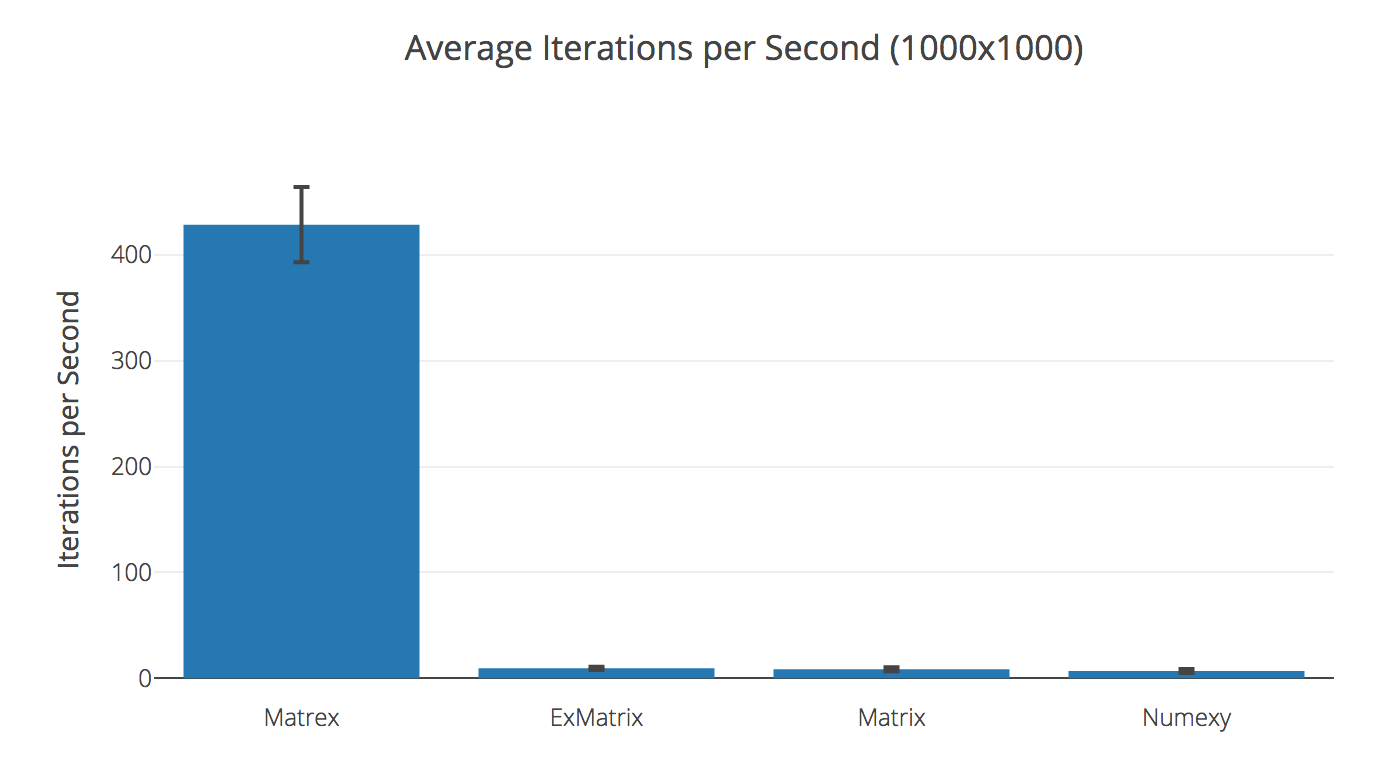## Example

Complete example of Matrex library at work: Linear regression on MNIST digits (Jupyter notebook)

## Visualization

Matrex implements `Inspect` protocol and looks nice in your console: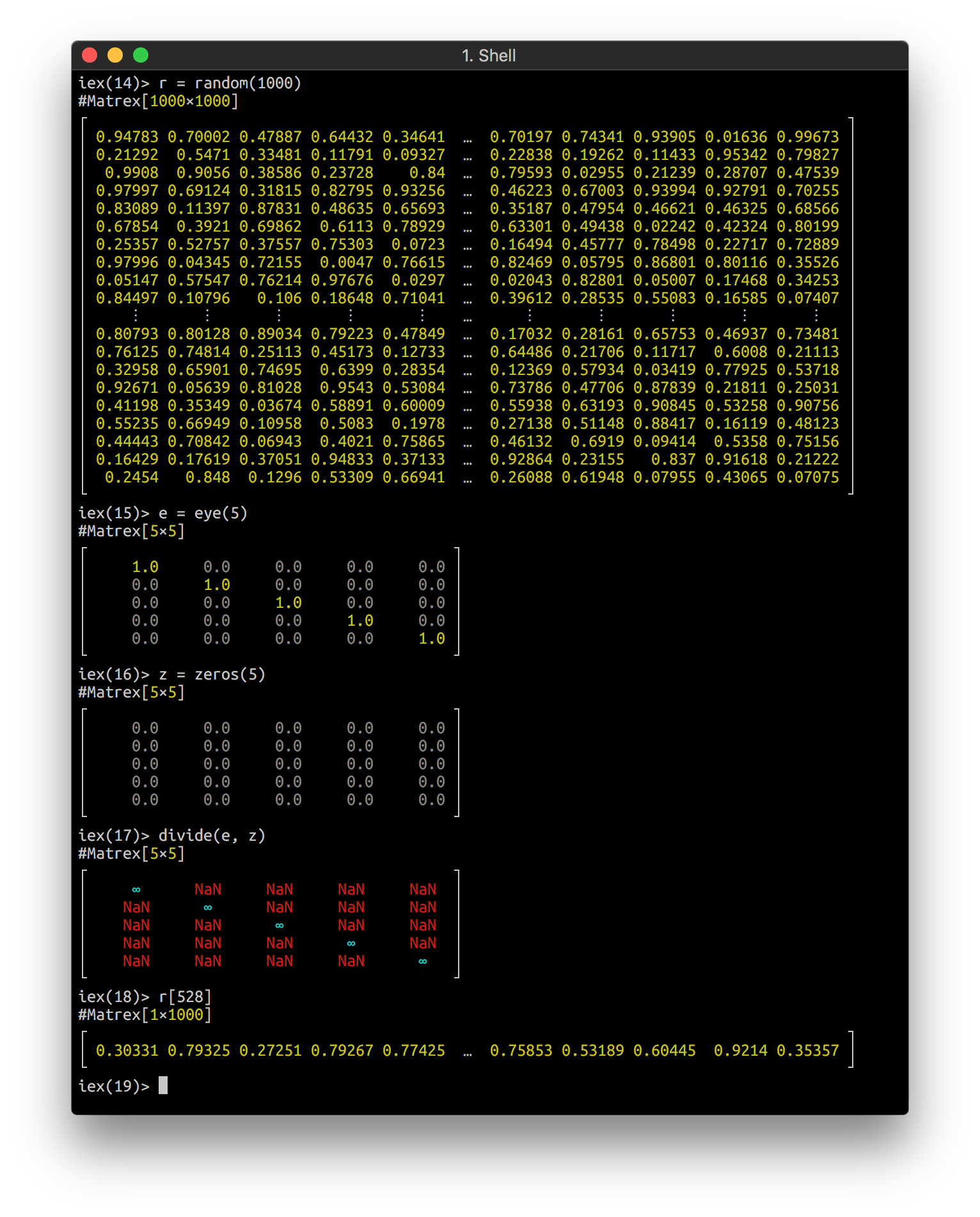It can even draw a heatmap of your matrix in console! Here is an animation of logistic regression training with Matrex library and some matrix heatmaps: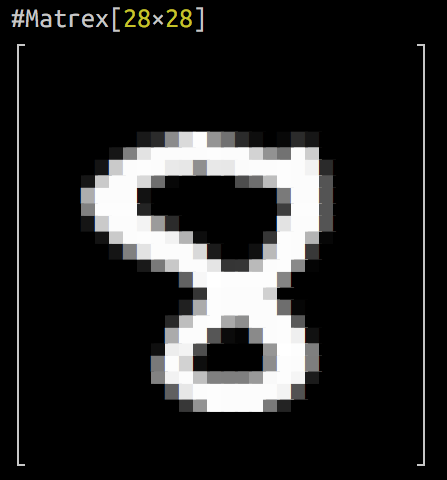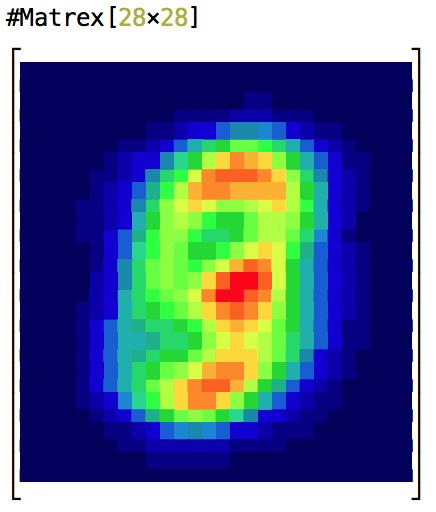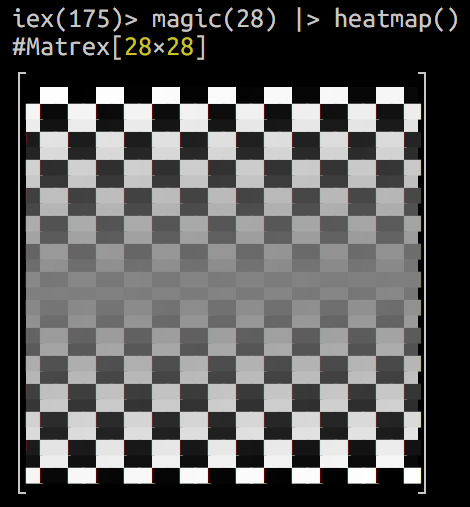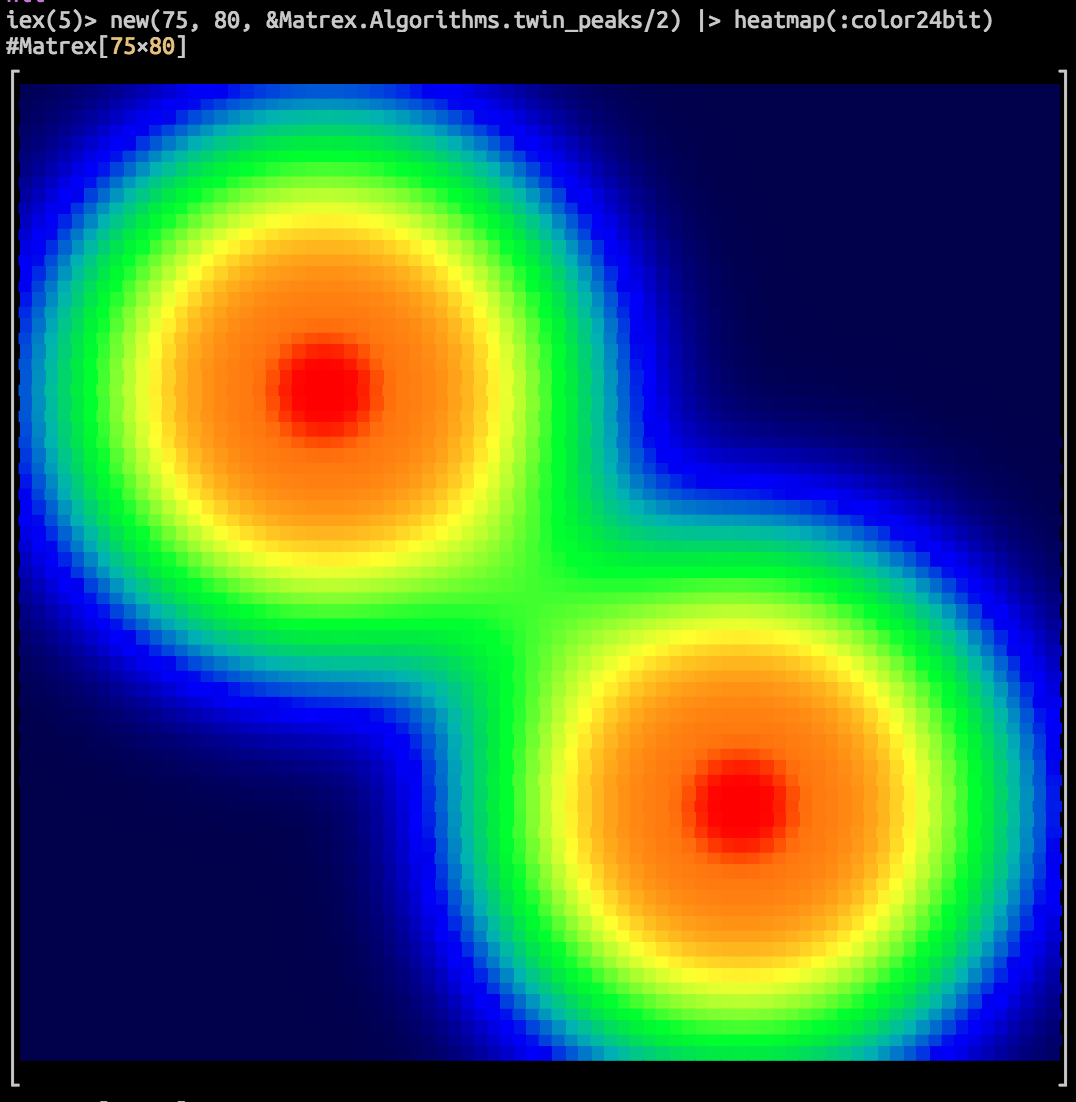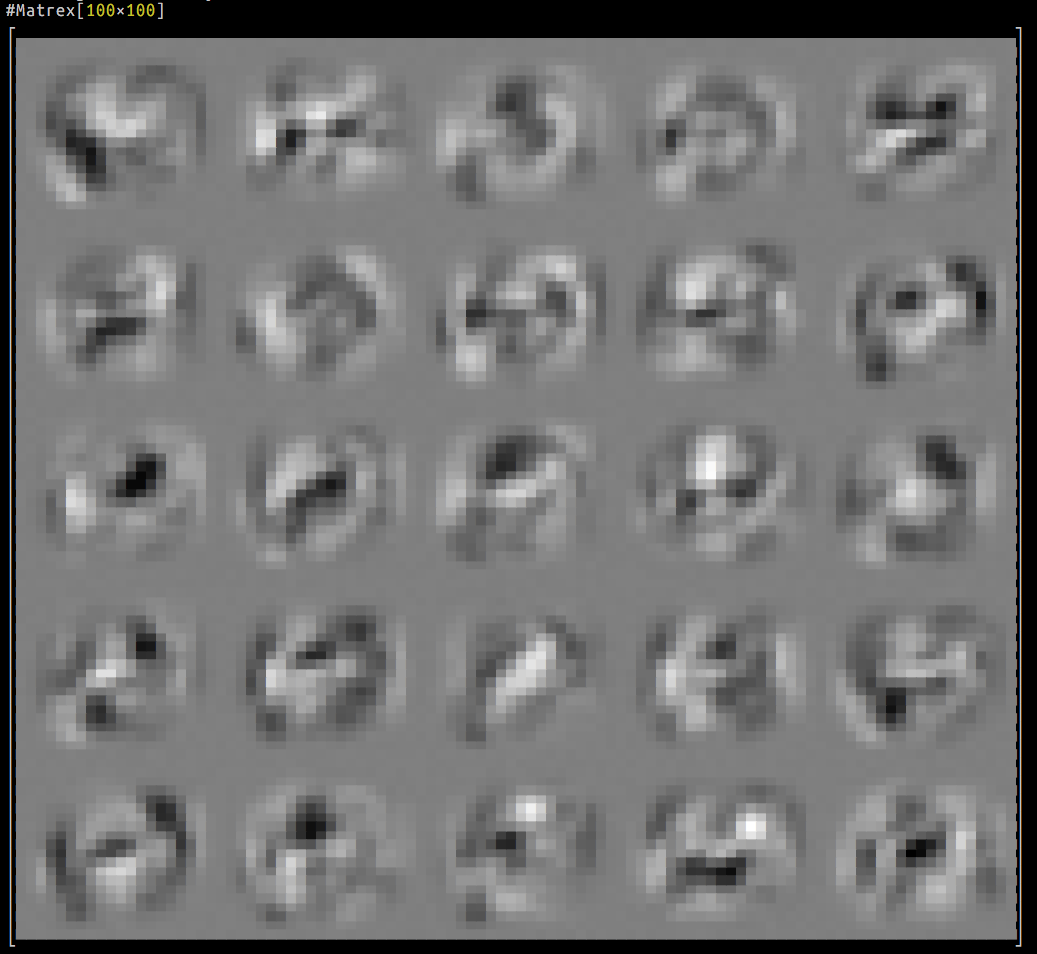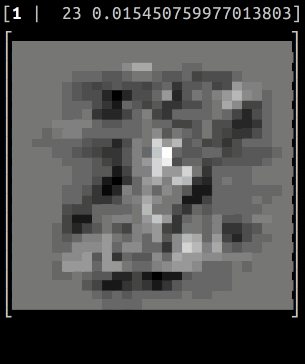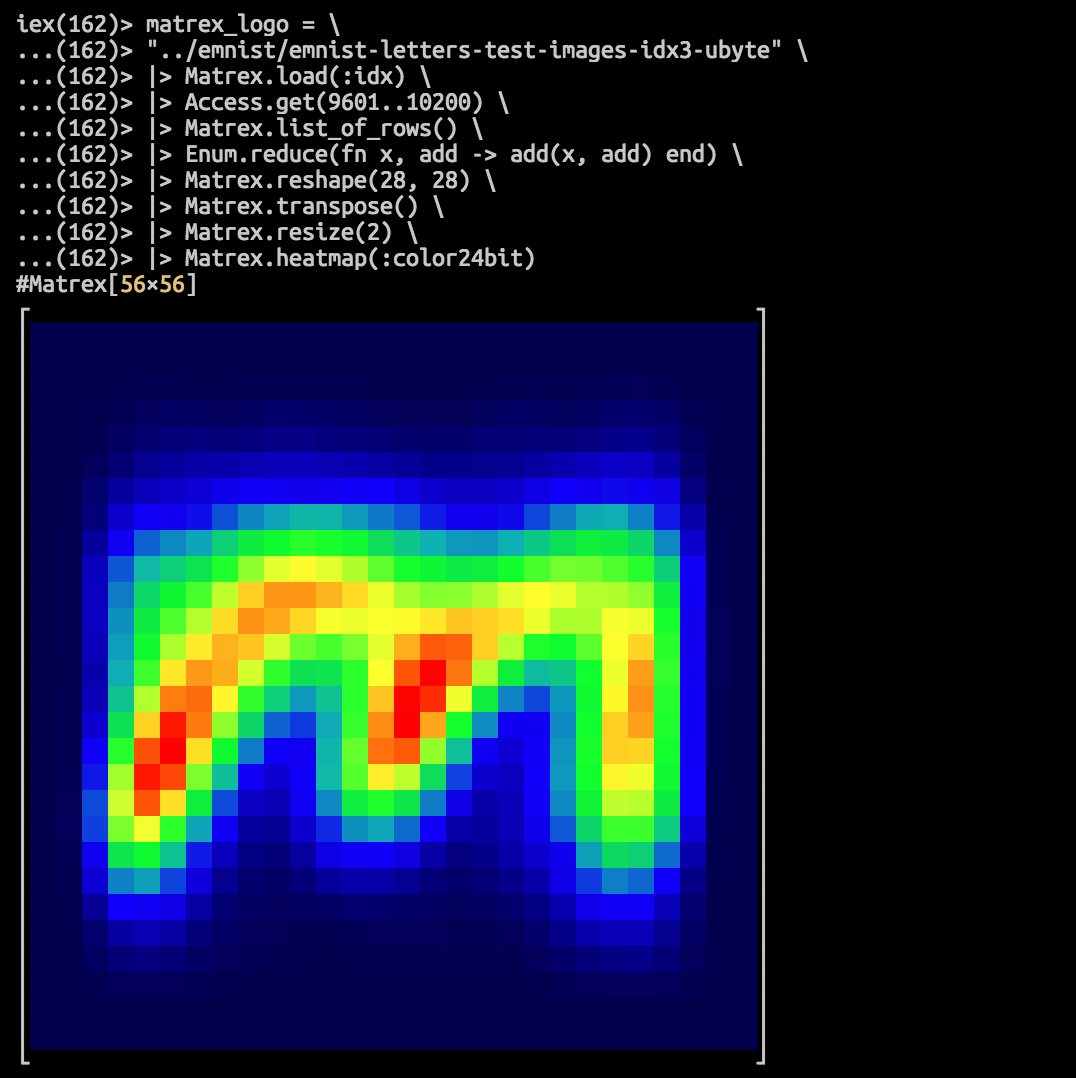## Installation

The package can be installed by adding `matrex` to your list of dependencies in `mix.exs`:

``````def deps do
[
{:matrex, "~> 0.6"}
]
end
``````

### MacOS

Everything works out of the box, thanks to Accelerate framework. If you encounter a compilation error

`native/src/matrix_dot.c:5:10: fatal error: 'cblas.h' file not found`

then make sure the XCode command-line tools are installed (`xcode-select --install`). If the error still not resolved, for MacOS Mojave, run `open /Library/Developer/CommandLineTools/Packages/macOS_SDK_headers_for_macOS_10.14.pkg` to restore /usr/include and /usr/lib.

On MacOS 10.15 this error can be solved with

`export CPATH=/Library/Developer/CommandLineTools/SDKs/MacOSX.sdk/usr/include/`

or with

`C_INCLUDE_PATH=/Library/Developer/CommandLineTools/SDKs/MacOSX.sdk/System/Library/Frameworks/Accelerate.framework/Frameworks/vecLib.framework/Headers mix compile`

### Ubuntu

You need to install scientific libraries for this package to compile:

``````> sudo apt-get install build-essential erlang-dev libatlas-base-dev
``````

### Windows

It will definitely work on Windows, but we need a makefile and installation instruction. Please, contribute.

### Choosing which BLAS to use

With the help of `MATREX_BLAS` environment variable you can choose which BLAS library to link with. It can take values `blas` (the default), `atlas`, `openblas` or `noblas`.

The last option means that you compile C code without any external dependencies, so, it should work anywhere with a C compiler place:

``````\$ mix clean
\$ MATREX_BLAS=noblas mix compile
``````

## Access behaviour

Access behaviour is partly implemented for Matrex, so you can do:

``````
iex> m = Matrex.magic(3)
#Matrex[3×3]
┌                         ┐
│     8.0     1.0     6.0 │
│     3.0     5.0     7.0 │
│     4.0     9.0     2.0 │
└                         ┘
iex> m
7.0
``````

Or even:

``````
iex> m[1..2]
#Matrex[2×3]
┌                         ┐
│     8.0     1.0     6.0 │
│     3.0     5.0     7.0 │
└                         ┘
``````

There are also several shortcuts for getting dimensions of matrix:

``````
iex> m[:rows]
3

iex> m[:size]
{3, 3}
``````

calculating maximum value of the whole matrix:

``````
iex> m[:max]
9.0
``````

or just one of it’s rows:

``````
iex> m[:max]
7.0
``````

calculating one-based index of the maximum element for the whole matrix:

``````
iex> m[:argmax]
8
``````

and a row:

``````
iex> m[:argmax]
3
``````

`Matrex.Operators` module redefines `Kernel` math operators (+, -, *, / <|>) and defines some convenience functions, so you can write calculations code in more natural way.

It should be used with great caution. We suggest using it only inside specific functions and only for increased readability, because using `Matrex` module functions, especially ones which do two or more operations at one call, are 2-3 times faster.

### Usage example

``````
def lr_cost_fun_ops(%Matrex{} = theta, { %Matrex{} = x, %Matrex{} = y, lambda } = _params)
when is_number(lambda) do
# Turn off original operators
import Kernel, except: [-: 1, +: 2, -: 2, *: 2, /: 2, <|>: 2]
import Matrex.Operators
import Matrex

m = y[:rows]

h = sigmoid(x * theta)
l = ones(size(theta)) |> set(1, 1, 0.0)

j = (-t(y) * log(h) - t(1 - y) * log(1 - h) + lambda / 2 * t(l) * pow2(theta)) / m

grad = (t(x) * (h - y) + (theta <|> l) * lambda) / m

end
``````

The same function, coded with module methods calls (2.5 times faster):

``````    def lr_cost_fun(%Matrex{} = theta, { %Matrex{} = x, %Matrex{} = y, lambda } = _params)
when is_number(lambda) do
m = y[:rows]

h = Matrex.dot_and_apply(x, theta, :sigmoid)
l = Matrex.ones(theta[:rows], theta[:cols]) |> Matrex.set(1, 1, 0)

regularization =
Matrex.dot_tn(l, Matrex.square(theta))
|> Matrex.scalar()
|> Kernel.*(lambda / (2 * m))

j =
y
|> Matrex.dot_tn(Matrex.apply(h, :log), -1)
|> Matrex.subtract(
Matrex.dot_tn(
Matrex.subtract(1, y),
Matrex.apply(Matrex.subtract(1, h), :log)
)
)
|> Matrex.scalar()
|> (fn
:nan -> :nan
x -> x / m + regularization
end).()

x
|> Matrex.dot_tn(Matrex.subtract(h, y))
|> Matrex.divide(m)

end
``````

## Enumerable protocol

Matrex implements `Enumerable`, so, all kinds of `Enum` functions are applicable:

``````
iex> Enum.member?(m, 2.0)
true

iex> Enum.count(m)
9

iex> Enum.sum(m)
45
``````

For functions, that exist both in `Enum` and in `Matrex` it’s preferred to use Matrex version, beacuse it’s usually much, much faster. I.e., for 1 000 x 1 000 matrix `Matrex.sum/1` and `Matrex.to_list/1` are 438 and 41 times faster, respectively, than their `Enum` counterparts.

You can save/load matrix with native binary file format (extra fast) and CSV (slow, especially on large matrices).

Matrex CSV format is compatible with GNU Octave CSV output, so you can use it to exchange data between two systems.

### Example

``````
iex> Matrex.random(5) |> Matrex.save("rand.mtx")
:ok
#Matrex[5×5]
┌                                         ┐
│ 0.05624 0.78819 0.29995 0.25654 0.94082 │
│ 0.50225 0.22923 0.31941  0.3329 0.78058 │
│ 0.81769 0.66448 0.97414 0.08146 0.21654 │
│ 0.33411 0.59648 0.24786 0.27596 0.09082 │
│ 0.18673 0.18699 0.79753 0.08101 0.47516 │
└                                         ┘
iex> Matrex.magic(5) |> Matrex.divide(Matrex.eye(5)) |> Matrex.save("nan.csv")
:ok
#Matrex[5×5]
┌                                         ┐
│    16.0     ∞       ∞       ∞       ∞   │
│     ∞       4.0     ∞       ∞       ∞   │
│     ∞       ∞      12.0     ∞       ∞   │
│     ∞       ∞       ∞      25.0     ∞   │
│     ∞       ∞       ∞       ∞       8.0 │
└                                         ┘
``````

## NaN and Infinity

Float special values, like `:nan` and `:inf` live well inside matrices, can be loaded from and saved to files. But when getting them into Elixir they are transferred to `:nan`,`:inf` and `:neg_inf` atoms, because BEAM does not accept special values as valid floats.

``````    iex> m = Matrex.eye(3)
#Matrex[3×3]
┌                         ┐
│     1.0     0.0     0.0 │
│     0.0     1.0     0.0 │
│     0.0     0.0     1.0 │
└                         ┘

iex> n = Matrex.divide(m, Matrex.zeros(3))
#Matrex[3×3]
┌                         ┐
│     ∞      NaN     NaN  │
│    NaN      ∞      NaN  │
│    NaN     NaN      ∞   │
└                         ┘

iex> n
:inf

iex> n
:nan
``````

## Creating Matrex logo from MNIST letters

``````    iex(166)> matrex_logo = \
...(166)> "../emnist/emnist-letters-test-images-idx3-ubyte" \